oomph::CRDoubleMatrixHelpers Namespace Reference

Namespace for helper functions for CRDoubleMatrices. More...

## Functions

void create_uniformly_distributed_matrix (const unsigned &nrow, const unsigned &ncol, const OomphCommunicator *const comm_pt, const Vector< double > &values, const Vector< int > &column_indices, const Vector< int > &row_start, CRDoubleMatrix &matrix_out)
Builds a uniformly distributed matrix. A locally replicated matrix is constructed then redistributed using OOMPH-LIB's default uniform row distribution. This is memory intensive thus should be used for testing or small problems only. More...

double inf_norm (const DenseMatrix< CRDoubleMatrix * > &matrix_pt)
Compute infinity (maximum) norm of sub blocks as if it was one matrix. More...

double gershgorin_eigenvalue_estimate (const DenseMatrix< CRDoubleMatrix * > &matrix_pt)
Calculates the largest Gershgorin disc whilst preserving the sign. Let A be an n by n matrix, with entries aij. For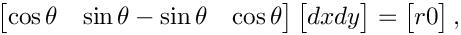let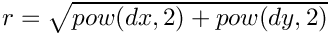be the sum of the absolute values of the non-diagonal entries in the i-th row. Let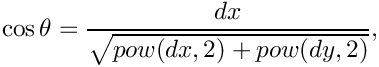be the closed disc centered at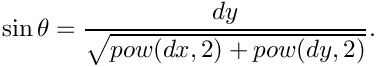with radius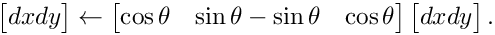, such a disc is called a Gershgorin disc. More...

void concatenate (const DenseMatrix< CRDoubleMatrix * > &matrix_pt, CRDoubleMatrix &result_matrix)
Concatenate CRDoubleMatrix matrices. The in matrices are concatenated such that the block structure of the in matrices are preserved in the result matrix. Communication between processors is required. If the block structure of the sub matrices does not need to be preserved, consider using CRDoubleMatrixHelpers::concatenate_without_communication(...). More...

void concatenate_without_communication (const Vector< LinearAlgebraDistribution * > &row_distribution_pt, const Vector< LinearAlgebraDistribution * > &col_distribution_pt, const DenseMatrix< CRDoubleMatrix * > &matrix_pt, CRDoubleMatrix &result_matrix)
Concatenate CRDoubleMatrix matrices. More...

void concatenate_without_communication (const Vector< LinearAlgebraDistribution * > &block_distribution_pt, const DenseMatrix< CRDoubleMatrix * > &matrix_pt, CRDoubleMatrix &result_matrix)
Concatenate CRDoubleMatrix matrices. This calls the other concatenate_without_communication(...) function, passing block_distribution_pt as both the row_distribution_pt and col_distribution_pt. This should only be called for block square matrices. More...

void deep_copy (const CRDoubleMatrix *const in_matrix_pt, CRDoubleMatrix &out_matrix)
Create a deep copy of the matrix pointed to by in_matrix_pt. More...

## Detailed Description

Namespace for helper functions for CRDoubleMatrices.

## ◆ concatenate()

 void oomph::CRDoubleMatrixHelpers::concatenate ( const DenseMatrix< CRDoubleMatrix * > & matrix_pt, CRDoubleMatrix & result_matrix )

Concatenate CRDoubleMatrix matrices. The in matrices are concatenated such that the block structure of the in matrices are preserved in the result matrix. Communication between processors is required. If the block structure of the sub matrices does not need to be preserved, consider using CRDoubleMatrixHelpers::concatenate_without_communication(...).

The matrix manipulation functions CRDoubleMatrixHelpers::concatenate(...) and CRDoubleMatrixHelpers::concatenate_without_communication(...) are analogous to the Vector manipulation functions DoubleVectorHelpers::concatenate(...) and DoubleVectorHelpers::concatenate_without_communication(...). Please look at the DoubleVector functions for an illustration of the differences between concatenate(...) and concatenate_without_communication(...).

Distribution of the result matrix: If the result matrix does not have a distribution built, then it will be given a uniform row distribution. Otherwise we use the existing distribution. This gives the user the ability to define their own distribution, or save computing power if a distribution has been pre-built.

NOTE: ALL the matrices pointed to by matrix_pt has to be built. This is not the case with concatenate_without_communication(...)

Definition at line 4349 of file matrices.cc.

## ◆ concatenate_without_communication() [1/2]

 void oomph::CRDoubleMatrixHelpers::concatenate_without_communication ( const Vector< LinearAlgebraDistribution * > & block_distribution_pt, const DenseMatrix< CRDoubleMatrix * > & matrix_pt, CRDoubleMatrix & result_matrix )

Concatenate CRDoubleMatrix matrices. This calls the other concatenate_without_communication(...) function, passing block_distribution_pt as both the row_distribution_pt and col_distribution_pt. This should only be called for block square matrices.

Definition at line 5885 of file matrices.cc.

## ◆ concatenate_without_communication() [2/2]

 void oomph::CRDoubleMatrixHelpers::concatenate_without_communication ( const Vector< LinearAlgebraDistribution * > & row_distribution_pt, const Vector< LinearAlgebraDistribution * > & col_distribution_pt, const DenseMatrix< CRDoubleMatrix * > & matrix_pt, CRDoubleMatrix & result_matrix )

Concatenate CRDoubleMatrix matrices.

The Vector row_distribution_pt contains the LinearAlgebraDistribution of each block row. The Vector col_distribution_pt contains the LinearAlgebraDistribution of each block column. The DenseMatrix matrix_pt contains pointers to the CRDoubleMatrices to concatenate. The CRDoubleMatrix result_matrix is the result matrix.

The result matrix is a permutation of the sub matrices such that the data stays on the same processor when the result matrix is built, there is no communication between processors. Thus the block structure of the sub matrices are NOT preserved in the result matrix. The rows are block-permuted, defined by the concatenation of the distributions in row_distribution_pt. Similarly, the columns are block-permuted, defined by the concatenation of the distributions in col_distribution_pt. For more details on the block-permutation, see LinearAlgebraDistributionHelpers::concatenate(...).

If one wishes to preserve the block structure of the sub matrices in the result matrix, consider using CRDoubleMatrixHelpers::concatenate(...), which uses communication between processors to ensure that the block structure of the sub matrices are preserved.

The matrix manipulation functions CRDoubleMatrixHelpers::concatenate(...) and CRDoubleMatrixHelpers::concatenate_without_communication(...) are analogous to the Vector manipulation functions DoubleVectorHelpers::concatenate(...) and DoubleVectorHelpers::concatenate_without_communication(...). Please look at the DoubleVector functions for an illustration of the differences between concatenate(...) and concatenate_without_communication(...).

Distribution of the result matrix: If the result matrix does not have a distribution built, then it will be given a distribution built from the concatenation of the distributions from row_distribution_pt, see LinearAlgebraDistributionHelpers::concatenate(...) for more detail. Otherwise we use the existing distribution. If there is an existing distribution then it must be the same as the distribution from the concatenation of row distributions as described above. Why don't we always compute the distribution "on the fly"? Because a non-uniform distribution requires communication. All block preconditioner distributions are concatenations of the distributions of the individual blocks.

/////////////// END OF PARANOID TESTS

Definition at line 5223 of file matrices.cc.

## ◆ create_uniformly_distributed_matrix()

 void oomph::CRDoubleMatrixHelpers::create_uniformly_distributed_matrix ( const unsigned & nrow, const unsigned & ncol, const OomphCommunicator *const comm_pt, const Vector< double > & values, const Vector< int > & column_indices, const Vector< int > & row_start, CRDoubleMatrix & matrix_out )

Builds a uniformly distributed matrix. A locally replicated matrix is constructed then redistributed using OOMPH-LIB's default uniform row distribution. This is memory intensive thus should be used for testing or small problems only.

Builds a uniformly distributed matrix. A locally replicated matrix is constructed then redistributed using OOMPH-LIB's default uniform row distribution. This is memory intensive thus should be used for testing or small problems only. The resulting matrix (mat_out) must not have been built.

Definition at line 3676 of file matrices.cc.

## ◆ deep_copy()

 void oomph::CRDoubleMatrixHelpers::deep_copy ( const CRDoubleMatrix *const in_matrix_pt, CRDoubleMatrix & out_matrix )
inline

## ◆ gershgorin_eigenvalue_estimate()

 double oomph::CRDoubleMatrixHelpers::gershgorin_eigenvalue_estimate ( const DenseMatrix< CRDoubleMatrix * > & matrix_pt )

Calculates the largest Gershgorin disc whilst preserving the sign. Let A be an n by n matrix, with entries aij. Forletbe the sum of the absolute values of the non-diagonal entries in the i-th row. Letbe the closed disc centered atwith radius, such a disc is called a Gershgorin disc.

We calculate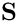and multiply by the sign of the diagonal entry.

The DenseMatrix of CRDoubleMatrices are treated as if they are one large matrix. Therefore the dimensions of the sub matrices has to "make sense", there is a paranoid check for this.

We calculateand multiply by the sign of the diagonal entry.

The DenseMatrix of CRDoubleMatrices are treated as if they are one large matrix. Therefore the dimensions of the sub matrices has to "make sense", there is a paranoid check for this.

Definition at line 4003 of file matrices.cc.

## ◆ inf_norm()

 double oomph::CRDoubleMatrixHelpers::inf_norm ( const DenseMatrix< CRDoubleMatrix * > & matrix_pt )

Compute infinity (maximum) norm of sub blocks as if it was one matrix.

Calculates the infinity (maximum) norm of a DenseMartrix of CRDoubleMatrices as if it was one large matrix. This avoids creating a concatenation of the sub-blocks just to calculate the infinity norm.

Definition at line 3731 of file matrices.cc.